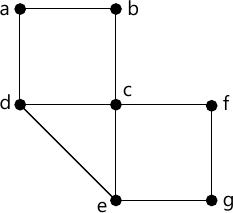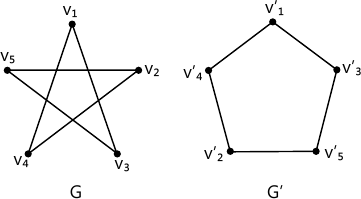MORE IN Discrete Structures
MU Computer Engineering (Semester 3)
Discrete Structures
May 2015
Total marks: --
Total time: --
INSTRUCTIONS
(1) Assume appropriate data and state your reasons
(2) Marks are given to the right of every question
(3) Draw neat diagrams wherever necessary

1 (a) Let A={a,b,c}. Show that (P(A),⊆) is poset. Draw its Hasse Diagram. P(A) is the power set of A.
5 M
1 (b) Find the generating function for the following finite sequence
i) 1, 2, 3, 4, ..... ii) 1, 1, 1, 1, 1, 1
5 M
1 (c) Is it possible to draw a tree with five vertices having degrees 1,1,2,2,4?
5 M
1 (d) Prove p∧ (p∨q) and (p∧q)∨(p∧r) are logically equivalent.
5 M

2 (a) If f:A→B be both one-to-one and onto, then prove that: f-1: B→A is also both one-to-one and onto.
4 M
2 (b) Let G be a set of rational number other than 1.let * be an operations on G defined by a*b*=a+b-ab for all a, b, ∈G. Prove that (G, *) is a group.
8 M
2 (c) Define Equivalence relation with an example. Let m be a positive integer other than 1. Show that the relation R={(a,b)|a=b(mod m)} i.e. m divides a-b is an equivalence relation on the set of integers.
8 M

3 (a) Show that the set of all divisors of 70 forms a lattice.
4 M
3 (b) Consider the (3,5) group encoding function defined by
 e(000)=00000 e(001)=00110 e(010)=01001 e(011)=01111 e(100)=10011 e(101)=10101 e(110)=11010 e(111)=11000

Decode the following words relative to a maximum likely decoding function.
i) 11001 ii) 01010 iii) 00111.
8 M
3 (c) Define Reflexive closure, Symmetric closure along with a suitable example. Let R be a relation on set S={a, b, c, d, e), gives as
R={(a.a), (a,d), (b,b), (c,d), (c,e), (d,e), (e,b), (e,e)}.
Find transitive closure using Warsnail's Algorithm.
8 M

4 (a) Determine Euler Cycle and path in graph shown below.4 M
4 (b) A survey of 500 television watches produced the following information:
285 watch footbal games, 195 watch hockey games, 115 watch basket ball games, 45 watch football and basketball games, 70 watch football and hockey games. 50 watch basketball and hockey games. 50 do not watch any three kinds of games. Find:
i) How many in the survey watch all 3 kinds of games?
ii) How many watch exactly one of the sport languages?
iii) Draw Venn Diagram showing results of the survey.
8 M
4 (c) Find the solution to the recurrence relation
an=6an-1+11an-2-6an-3 given a0=20, a1=5 and a2=15.
8 M

5 (a) Show that if every element in a group its own inverse, then the group must be abelian.
4 M
5 (b) Explain Pigeonhole principle and Extended Pigeonhole Principle. Show that if 7 colors are used to paint 50 bicycles, at least 8 bicycles will be of same color.
8 M
5 (c) (i) Prove by mathematical induction xn-yn is divisible by x-y.
4 M
5 (c) (ii) Consider the group G={1, 2, 3, 4, 5, 6} under multiplication modulo 7.
a) Find multiplication table of G
b) Find inverse of every element.
4 M

6 (a) Show that A-(B-C)=(A-B)∪(A∩B∩C).
4 M
6 (b) "$Let \ H=\begin{vmatrix}1 &0 &0 \\0 &1 &1 \\1 &1 &1 \\1 &0 &0 \\0 &1 &0 \\0 &0 &1 \end{vmatrix}$ Be a parity check matrix. Determine the group code eH B3→B6."
8 M
6 (c) Determine if following graphs g and G' are isomorphic or not.8 M

More question papers from Discrete Structures